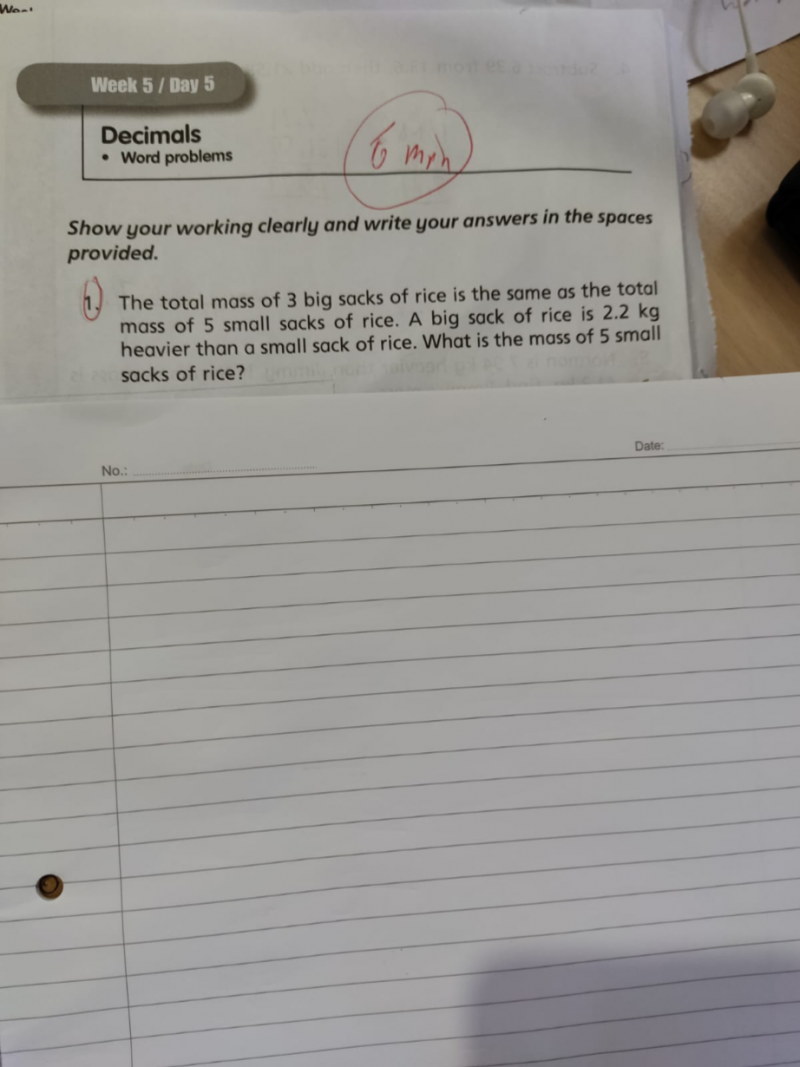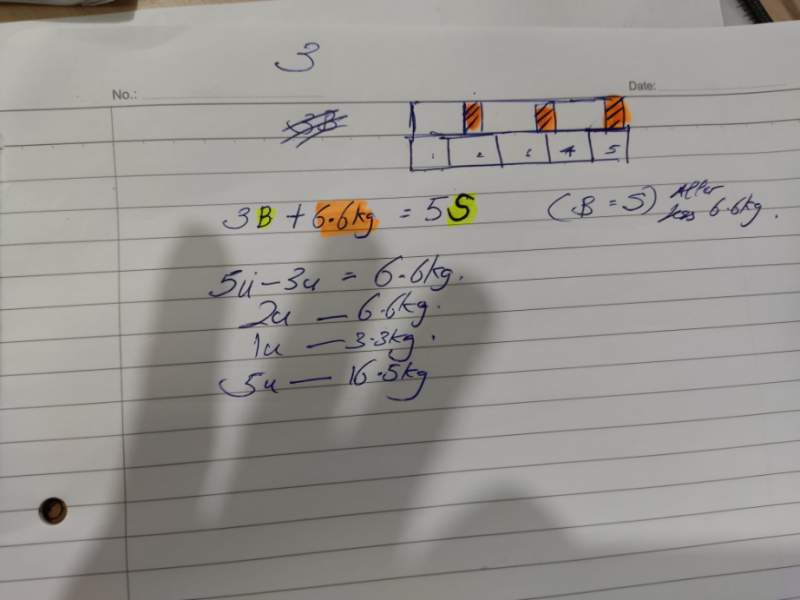# QuestionGuys, I need help with this.

i.e. Draw Diagram to explain to my kids.

I am new to this forum.

Didn’t know that nowadays Math is getting so chim.

Your diagram is conceptually ok (if your child can understand) but mathematically inaccurate so probably easier to draw it like that below if you want to explain why B = S = u.  In other words, from your diagram, it is hard to see that B = S = u.   Strictly speaking, if B stands for big sack and S for small sack, then B = S + 2.2.    See below for how to arrive at the same answer.

SS     [  u  ][  u  ][  u  ][  u  ][  u  ]

BS     [  u  ][  u  ][  u  ][     6.6     ]

2u = 6.6

u = 3.3

Hence, 3.3 x 5 = 16.5kg

Continuing from B = S + 2.2 above ,   then let S = u, B = u + 2.2   (that’s why B <> S)

3B = 5S

3(u+2.2) = 5u

3u + 6.6 = 5u   gives u = 3.3 and mass of 5 small sacks = 16.5kg

0 Replies 2 Likes ✔Accepted Answer

Big sack                 [                  ][                   ][                 ]    <—–original 3 units

<—-5u—–> <—-5u—–> <—-5u—–>

Small sack             [  3u  ] [   3u   ] [  3u ] [   3u  ] [  3u   ]    <——original 5 units

<3u ><2.2>

<——————- 15u ——————–>

For them to be compared, make both of them into 15 equal units as total,

this means each big sack = 5u and each small sack  = 3u

So, 5u-3u = 2u = 2.2   => u = 1.1

Mass of each small sack = 1.1 x 3 = 3.3

Hence mass of 5 small sacks = 3.3 x 5 = 16.5 kg   (also = 3 big sacks)Your answer is correct as per the worksheet answer. But sadly it is very profound for me, seems I have lost touch lol.

Lol, for me the current level for Math for me is more difficult than earning money in equities.

Apology, I have some more queries.

I have done something with the diagram. Is it correct to explain to my child using this diagram?

Your diagram is conceptually ok (if your child can understand) but mathematically inaccurate so probably easier to draw it like that below if you want to explain why B = S = u.  In other words, from your diagram, it is hard to see that B = S = u.   Strictly speaking, if B stands for big sack and S for small sack, then B = S + 2.2.    See below for how to arrive at the same answer.

SS     [  u  ][  u  ][  u  ][  u  ][  u  ]

BS     [  u  ][  u  ][  u  ][     6.6     ]

2u = 6.6

u = 3.3

Hence, 3.3 x 5 = 16.5kg

Continuing from B = S + 2.2 above ,   then let S = u, B = u + 2.2   (that’s why B <> S)

3B = 5S

3(u+2.2) = 5u

3u + 6.6 = 5u   gives u = 3.3 and mass of 5 small sacks = 16.5kg

0 Replies 2 Likes ✔Accepted Answer